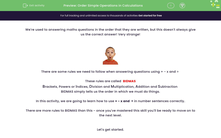# Order Simple Operations in Calculations

In this worksheet, students will begin to earn how to use the rules of BIDMA/BODMAS.Key stage:  KS 2

Curriculum topic:   Number: Addition, Subtraction, Multiplication and Division

Curriculum subtopic:   Carry Out Calculations Using Order of Operations

Difficulty level:#### Worksheet Overview

We're used to answering maths questions in the order that they are written, but this doesn't always give us the correct answer! Very strange!There are some rules we need to follow when answering questions using + - x and ÷

These rules are called  BIDMAS

Brackets, Powers or Indices, Division and Multiplication, Addition and Subtraction

BIDMAS simply tells us the order in which we must do things.

In this activity, we are going to learn how to use + - x and  ÷ in number sentences correctly.

There are more rules to BIDMAS than this - once you've mastered this skill you'll be ready to move on to the next level.

Let's get started.If we have a number sentence that includes both addition or subtraction, and multiplication or division, we do the multiplication/division first (even if it is at the end of the question).

6 + 9 ÷ 3 = 6 + 3 = 9 (divide first)

7 + 8 x 2 = 7 + 16 = 23 (multiply first)

12 ÷ 4 + 10 = 3 + 10 = 13 (divide first)

3 x 7 - 6 = 21 - 6 = 15 (multiply first)

If we have a question that has just multiplication and division, we do them in the order in which they are written.

If we have a question that has just addition and subtraction, we do them in the order in which they are written (left to right).

15 + 6 - 3 = 18

15 - 3 + 12 = 24

4 x 9 ÷ 3 = 12

27 ÷ 3 x 2 = 18Now, why don't you have a go at answering some similar questions?

Come back and have another look at this page if you get stuck by clicking on the red help button on the screen.

Good luck!

### What is EdPlace?

We're your National Curriculum aligned online education content provider helping each child succeed in English, maths and science from year 1 to GCSE. With an EdPlace account you’ll be able to track and measure progress, helping each child achieve their best. We build confidence and attainment by personalising each child’s learning at a level that suits them.

Get started••••